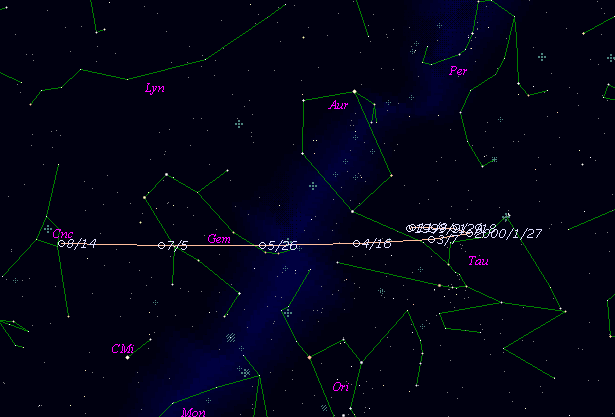# \$B%j%K%"WB@1(B

165P/LINEAR (2000)###\$B%W%m%U%#!<%k(B

 \$BId9f(B 165P/2000 B4 \$BH/8+F|(B 2000\$BG/(B1\$B7n(B29\$BF|(B \$BH/8+8wEY(B 19.4\$BEy(B \$BH/8+ Lincoln Laboratory Near-Earth Asteroid Research project

###\$B50F;MWAG(B

```   The following improved orbital elements by Kenji Muraoka, are
from 109 observations 2000 Jan. 2 to 2001 Mar. 23, perturbations
by 9 Planets, Moon and 5 minor planets were taken into account.
The mean residual is +/- 0.54 arc seconds.

Epoch  =  2000 June 25.0  TT       JDT = 2451720.5
T  =  2000 June 14.56645       +/- 0.09317 (m.e.) TT
Peri. =  126.13348                +/- 0.01531
Node  =    0.62668                +/- 0.01622   (2000.0)
Incl. =   15.90933                +/- 0.00006
q  =    6.8295638              +/- 0.0005703 AU
e  =    0.6207126              +/- 0.0000218
a  =   18.0063007              +/- 0.0010919 AU
n  =    0.01289934             +/- 0.00000117
P  =   76.408                  +/- 0.0069498  years
(+/- 2.54 days)
```

###\$B@1?^(B###\$B8wEYJQ2=(B

```        m1 = 7.0 + 5 log\$B&\$(B + 10.0 log r
```##### \$B50F;MWAG\$OB<2,7r<#;a\$N7W;;\$K\$h\$k\$b\$N\$G\$9!#(B \$B@1?^\$O(B StellaNavigator Ver.2.0 for Windows (\$B%"%9%H%m%"!<%D(B \$BJTCx(B / \$B%"%9%-!<=PHG6I4)(B) \$B\$G:n@.\$7\$?\$b\$N\$G\$9!#(B \$B8wEY%0%i%U\$O(BComet for Windows\$B\$G:n@.\$7\$?\$b\$N\$G\$9!#(B